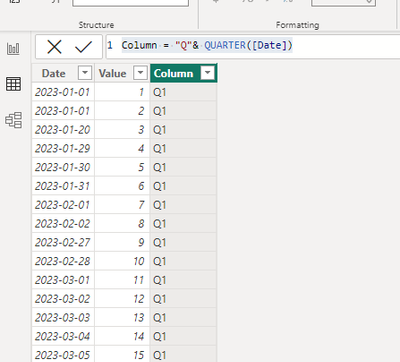cancel
Showing results for
Did you mean:Resolver I

## Bar chart with marking for Quarters

I am representing the annual target on a bar chart and comparing with the completion data every month during the year. The monthly data is cumulative - that is April data consists of the sum of four months in the year. That is basically two bars, annual target on the top and YTD month-end data below that bar.  I am not using any time scale on the chart. I only indicate the YTD (year to date) month in a card above the chart. The two bars represent two numbers.

I want the top bar, representing the annual target, to be marked Q1,Q2 and Q3 at equal intervals. Which is the simplest way to achieve that?

1 ACCEPTED SOLUTIONCommunity Support

Hi, @kkanda

Sorry I misunderstood you, from your description you want to divide one of your values into 4 equal parts.

This is my test data:We can create three measures as the constant line.

``Q1 = [Total]/4``
``Q2 = [Total]/2``
``Q3 = ([Total]/4)*3``

Then we can configure the measure on the "Constant Line":Best Regards,

Aniya Zhang

If this post helps, then please consider Accept it as the solution to help the other members find it more quickly

4 REPLIES 4Resolver I

Thank you. This has helped me in dividing the target value to 4 parts.Resolver I

Probably I was not clear what I am looking for. In the attached chart, 1064 is the annual target and 250 is the completed number up to end of April. The X- Axis is a number and there is no Y-Axis. I want the bar representing 1064 to be divided in 4 equal parts marking as "Q1", "Q2", "Q3" and "Q4".Community Support

Hi, @kkanda

Sorry I misunderstood you, from your description you want to divide one of your values into 4 equal parts.

This is my test data:We can create three measures as the constant line.

``Q1 = [Total]/4``
``Q2 = [Total]/2``
``Q3 = ([Total]/4)*3``

Then we can configure the measure on the "Constant Line":Best Regards,

Aniya Zhang

If this post helps, then please consider Accept it as the solution to help the other members find it more quicklyCommunity Support

Hi, @kkanda

According to your description, you have calculated the value of YTD, do you want to divide the value of YTD into quarters?

If this , you can just create a calculated column in your table:

``Column = "Q"& QUARTER([Date])``Then you can the column to the dimension field and your measure on the value , then we can get this:If this method does not meet your needs, you can provide us with your special sample data and the desired output sample data in the form of tables, so that we can better help you solve the problem. (You can also upload you sample .pbix [without sensitive data] to the OneDrive and share with the OneDrive link to me ! )

Thank you for your time and sharing, and thank you for your support and understanding of PowerBI!

Best Regards,

Aniya Zhang

If this post helps, then please consider Accept it as the solution to help the other members find it more quickly